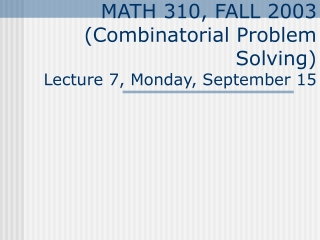Download PresentationMATH 310, FALL 2003 (Combinatorial Problem Solving) Lecture 7, Monday, September 15

# MATH 310, FALL 2003 (Combinatorial Problem Solving) Lecture 7, Monday, September 15 - PowerPoint PPT PresentationDownload Presentation## MATH 310, FALL 2003 (Combinatorial Problem Solving) Lecture 7, Monday, September 15

- - - - - - - - - - - - - - - - - - - - - - - - - - - E N D - - - - - - - - - - - - - - - - - - - - - - - - - - -
##### Presentation Transcript

1. MATH 310, FALL 2003(Combinatorial Problem Solving)Lecture 7, Monday, September 15

2. Chapter 1 Review • Homework (MATH 310#3M): • Read Supplement I (pp 46-48). Write down a list of all newly introduced terms (printed in boldface or italic) • Do Ch.1 Supplement II. Exercises : 4,14,16,20,28,30,36. • Volunteers: • ____________ • ____________ • Problem: 30.

3. Example: n = 3. There are 8 binary sequences: 000 001 010 011 100 101 110 111 There are 2n binary sequences of length n. An ordering of 2n binary sequences with the property that any two consecutive elements differ in exactly one position is called a Gray code. Example 5: Gray Code

4. Hypercube • Thegraph with one vertex for each n-digit binary sequence and an edge joining vertices that correspond to sequences that differ in just one position is called an n-dimensional cube or hypercube. • v = 2n • e = n 2n-1

5. 4-dimensional Cube. 0110 0010 0111 1110 0011 1010 1011 1111 0001 1101 1001 0000 0100 1100 1000

6. 4-dimensional Cube and a Famous Painting by Salvador Dali • Salvador Dali (1904 – 1998) produced in 1954 the Crucifixion (Metropolitan Museum of Art, New York) in which the cross is a 3-dimensional net of a 4-dimensional hypercube.

7. Gray Code - Revisited 010 011 • A Hamilton path in the hypercube produces a Gray code. 111 110 100 101 001 000

8. 2.3 Graph Coloring • Homework (MATH 310#3W): • Read 2.4. Write down a list of all newly introduced terms (printed in boldface or italic) • Do Exercises 2.3: 2,4,6,7,12,14,18 • Volunteers: • ____________ • ____________ • Problem: 7. • On Monday you will also turn in the list of all new terms with the following marks • + if you think you do not need the definition on your cheat sheet, • check (if you need just the term as a reminder), • - if you need more than just the definition to understand the term.

9. Coloring and Chromatic Number • A coloring of a graph G assigns colors to the vertices of G so that adjacent vertices are given different colors. • The minimal number of colors required to color a given graph is called the chromatic number of a graph.

10. Example 1: Simple Graph Coloring • Find the chromatic number of the graph on the left.